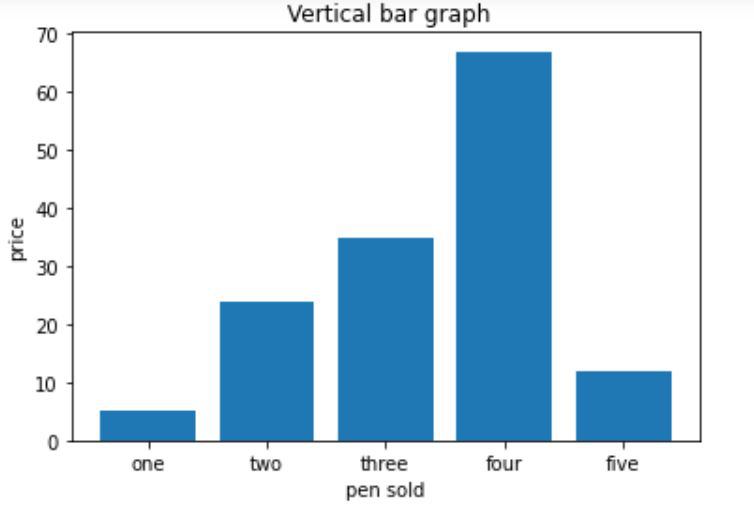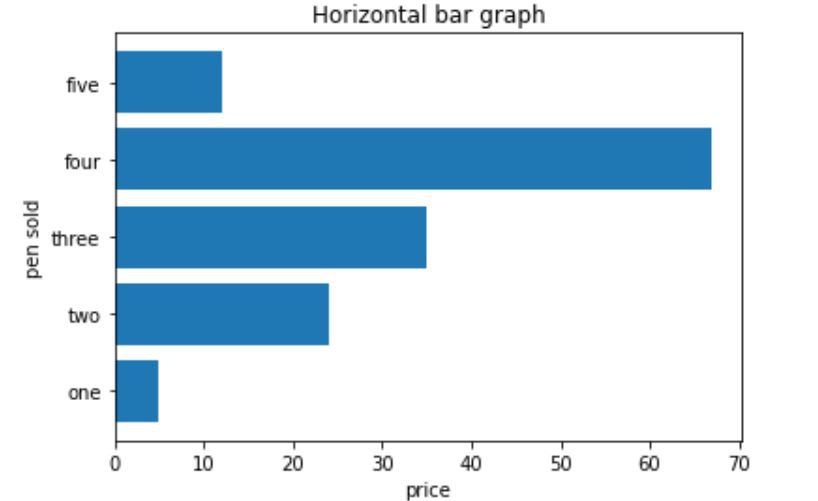# Draw a horizontal bar chart with Matplotlib

• Last Updated : 25 Aug, 2021

Matplotlib is the standard python library for creating visualizations in Python. Pyplot is a module of Matplotlib library which is used to plot graphs and charts and also make changes in them. In this article, we are going to see how to draw a horizontal bar chart with Matplotlib.

### Creating a vertical bar chart

Approach:

Attention geek! Strengthen your foundations with the Python Programming Foundation Course and learn the basics.

To begin with, your interview preparations Enhance your Data Structures concepts with the Python DS Course. And to begin with your Machine Learning Journey, join the Machine Learning - Basic Level Course

• Importing matplotlib.pyplot as plt
• Creating list x for discrete values on x-axis
• Creating list y  consisting only numeric data for discrete values on y-axis
• Calling plt.bar() function with parameters x,y as plt.bar(x,y)
• Setting x_label() and y_label()
• Setting title() for our bar chart
• Calling plt.show() for visualizing our chart

Below is the implementation:

## Python3

 `import` `matplotlib.pyplot as plt`   `x``=``[``'one'``, ``'two'``, ``'three'``, ``'four'``, ``'five'``]`   `# giving the values against` `# each value at x axis` `y``=``[``5``, ``24``, ``35``, ``67``, ``12``] ` `plt.bar(x, y)`   `# setting x-label as pen sold` `plt.xlabel(``"pen sold"``) `   `# setting y_label as price` `plt.ylabel(``"price"``)   ` `plt.title(``" Vertical bar graph"``)` `plt.show()`

Output:### Creating a horizontal bar chart

Approach:

• Importing matplotlib.pyplot as plt
• Creating list y   for discrete values on y-axis
• Creating list x  consisting only numeric data for discrete values on x-axis
• Calling plt.barh() function with parameters y,x as plt.barh(y,x)
• Setting x_label() and y_label()
• Setting title() for our bar chart
• Calling plt.show() for visualizing our chart

Below is the implementation:

## Python3

 `import` `matplotlib.pyplot as plt` `y``=``[``'one'``, ``'two'``, ``'three'``, ``'four'``, ``'five'``]`   `# getting values against each value of y` `x``=``[``5``,``24``,``35``,``67``,``12``] ` `plt.barh(y, x)`   `# setting label of y-axis` `plt.ylabel(``"pen sold"``)`   `# setting label of x-axis` `plt.xlabel(``"price"``) ` `plt.title(``"Horizontal bar graph"``)` `plt.show()`

Output:My Personal Notes arrow_drop_up
Recommended Articles
Page :